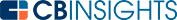VC GLOSSARY

# What is the Confidence Interval Excel Function?

A confidence interval is a percentage that indicates the percent of time that the results of an experiment will fall within the margin of error. The confidence interval Excel function is a built-in function that enables users to more easily calculate a confidence interval for a set of data.

As a field, statistics is not exactly like the pure sciences, and the results of statistical modeling will vary according to the data set used. Scientific endeavors need repeatability to verify claims, and statistical models do not offer precise results when repeated over and over with different populations. Confidence levels and confidence intervals are used for this purpose.

## Confidence level

A confidence interval is generally represented as a percentage figure. Let’s see what a confidence level of 95% signifies. When the same experiment is carried out using different populations, the results will fall within the margin of error 95% of the time.

If that does not happen, it means that the population used for the initial calculation was not a representative sample. Commonly used confidence intervals are 90%, 95%, and 99%. For the purposes of calculating confidence intervals, α is used. α is the inverse of the confidence interval.

For a confidence interval of 90%, α=0.10.

For a confidence interval of 95%, α=0.05.

For a confidence interval of 99%, α=0.01.

α is called the significance level.

## Confidence interval

A confidence interval is the range including the margin of error. Suppose the mean of a sample is 200 and the margin of error is 5. The confidence interval will be the range from 195 to 205, or 200 ± 5. Suppose the confidence level is 95%. That means when the experiment is repeated with different populations, the mean will fall between 195 and 205 in 95% of cases.

## Confidence interval in Excel

The computation of a confidence interval taking the t-distribution and Z-value takes effort. But Excel offers a simple function to calculate the confidence interval for a population with a known mean and standard deviation. The confidence level required is presented as α.

The function in Excel that is used to calculate confidence interval is:

=CONFIDENCE()

The function including the parameters required becomes:

=CONFIDENCE(alpha, standard_dev, size)

Here:

• alpha is the confidence level required represented as α,
• standard_dev is the standard deviation of the population, and
• size is the sample size of the population under consideration.

The result of CONFIDENCE() is the margin of error. To find the confidence interval, use the following:

CONFIDENCE INTERVAL = MEAN ± CONFIDENCE

Here is an example in Excel. The population size is 500 with a mean of 54 and a standard deviation of 1.6. The confidence level required is 99%. The corresponding significance level, or α, is 0.01.The result is:The margin of error is 0.18431134.

Confidence interval = 54 ± 0.18431134

The results will fall within 53.81568866 and 54.18431134 in 99% of instances when repeating the calculation multiple times for different populations.

The confidence interval formula in Excel is a function that allows you to more easily calculate the confidence interval for a set of data. The confidence interval indicates how frequently the results will fall within a specified range when repeating an experiment or calculation.

You may also like# Corporate InnovationCorporate StrategyCorporate Venture CapitalVenture CapitalLove Notes